Continuity equationThis article is a 'stub' page, it has no, or next to no, content. It is here at the moment to help form part of the structure of SklogWiki. If you add sufficient material to this article then please remove the {{Stub-general}} template from this page.

The continuity equation expresses the conservation of mass. It is a direct consequence of Gauss theorem.

If the mass enclosed in a region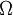$\Omega$ is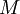$M$, by definition of mass density$\rho$: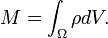$M=\int_\Omega \rho dV .$

The net loss of matter in this region must be caused by an outward flow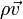$\rho \vec{v}$ across its boundary: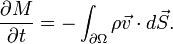$\frac{\partial M}{\partial t}= - \int_{\partial\Omega} \rho \vec{v} \cdot d\vec{S} .$

According to Gauss theorem,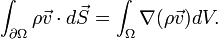$\int_{\partial\Omega} \rho \vec{v} \cdot d\vec{S} = \int_\Omega \nabla( \rho \vec{v} ) dV .$

Since the region is a general one, and it does not change with time, the resulting equation is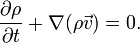$\frac{\partial \rho}{\partial t} + \nabla (\rho \vec{v}) =0 .$

As a direct consequence an incompressible fluid, with constant$\rho$, implies a solenoidal velocity field: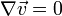$\nabla \vec{v} =0$.